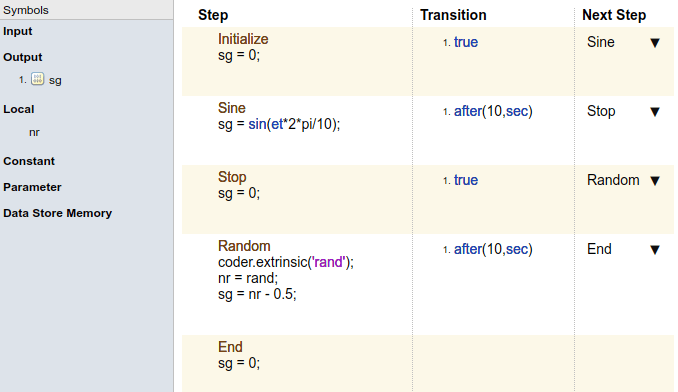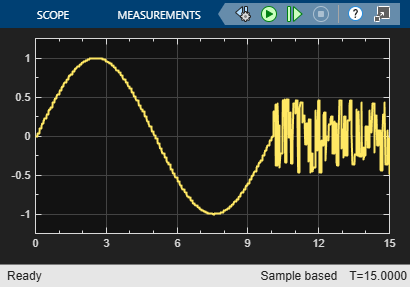## Generate Test Signals

In the Test Sequence block, you can generate signals to use for testing. First, define an output data symbol using the Data Symbols pane, and then use that output name with a signal generation function in a test step. For information on adding symbols, see Manage Input, Output, and Data Objects. For an example that shows how to implement signal functions in a Test Sequence block, see Sinusoidal and Random Number Functions in Test Sequences

### Signal Generation Functions

The following table lists common functions you can use in the Test Sequence block to create test signals, random number values, and natural exponents. It also describes the `latch` function, which saves and returns a specific value evaluated within a test sequence step. For more information about each function, click its name in the first column.

Some signal generation functions use the temporal operator `et`, which is the elapsed time of the test step in seconds. For additional operators related to `et` that you can use in test sequence steps, see Temporal Operators.

Note

Scaling, rounding, and other approximations of argument values can affect function outputs.

FunctionSyntaxDescriptionExample
`sin``sin(x)`

Returns the sine of `x`, where `x` is in radians.

A sine wave with a period of 10 sec:

`sin(et*2*pi/10)`
`cos``cos(x)`

Returns the cosine of `x`, where `x` is in radians.

A cosine wave with a period of 10 sec:

`cos(et*2*pi/10)`
square`square(x)`

Square wave output with a period of `1` and range `–1` to `1`.

Within the interval `0 <= x < 1`, `square(x)` returns the value `1` for `0 <= x < 0.5`and `–1` for ```0.5 <= x < 1```.

`square` is not supported in Stateflow® charts.

Output a square wave with a period of `10` sec:

`square(et/10)`
sawtooth`sawtooth(x)`

Sawtooth wave output with a period of `1` and range `–1` to `1`.

Within the interval `0 <= x < 1`, `sawtooth(x)` increases.

`sawtooth` is not supported in Stateflow charts.

Output a sawtooth wave with a period of `10` sec:

`sawtooth(et/10)`
triangle`triangle(x)`

Triangle wave output with a period of `1` and range `–1` to `1`.

Within the interval `0 <= x < 0.5`, `triangle(x)` increases.

`triangle` is not supported in Stateflow charts.

Output a triangle wave with a period of `10` sec:

`triangle(et/10)`
ramp`ramp(x)`

Ramp signal of slope `1`, returning the value of the ramp at time `x`.

`ramp(et)` effectively returns the elapsed time of the test step.

`ramp` is not supported in Stateflow charts.

Ramp one unit for every 5 seconds of test step elapsed time:

`ramp(et/5)`
heaviside`heaviside(x)`

Heaviside step signal, returning `0` for `x < 0` and `1` for ```x >= 0```.

`heaviside` is not supported in Stateflow charts.

Output a heaviside signal after `5` seconds:

`heaviside(et-5)`
`exp``exp(x)`

Returns the natural exponential function, ${e}^{x}$.

An exponential signal progressing at one tenth of the test step elapsed time:

`exp(et/10)`
`rand``rand`

Uniformly distributed pseudorandom values

Generate new random values for each simulation by declaring `rand` extrinsic with `coder.extrinsic`. Assign the random number to a local variable. For example:

```coder.extrinsic('rand') nr = rand sg = a + (b-a)*nr```
`randn``randn`

Normally distributed pseudorandom values

Generate new random values for each simulation by declaring `randn` extrinsic with `coder.extrinsic`. Assign the random number to a local variable. For example:

```coder.extrinsic('randn') nr = randn sg = nr*2```
latch`latch(x)`

Saves the value of `x` at the first time `latch(x)` evaluates in a test step, and subsequently returns the saved value of `x`. Resets the saved value of `x` when the step exits. Reevaluates `latch(x)` when the step is next active.

`latch` is not supported in Stateflow charts.

Latch `b` to the value of `torque`:

`b = latch(torque)`

### Sinusoidal and Random Number Functions in Test Sequences

This example shows how to produce a sine and a random number test signal in a Test Sequence block.

If you recreate this test sequence, before running it, set the `nr` symbol size and type. Hover over the `nr` symbol and click its Edit icon to open the Data Inspector. Set the `Size` to `1` and the `Type` to `double`.

The step `Sine` outputs a sine wave with a period of 10 seconds, specified by the argument `et*2*pi/10`. The step `Random` outputs a random number in the interval `-0.5 to 0.5`.The test sequence produces signal `sg`.